# UCOSUB

ModelingCalculates a constraint value and its derivatives for a user defined constraint element. User- defined constraints are typically used in conjunction to define a broad range of holonomic and non-holonomic constraints. A typical example is the so called "curve of pursuit" constraint, where one particle is chasing another particle.

## Use

User-defined surface calling a UCOSUB for the calculation of a user constraint:

<Constraint_UserConstr
id                  = "1"
usrsub_param_string = "USER(1,31,301,1,350,302)"
usrsub_dll_name     = "NULL">
</Constraint_UserConstr>

## Format

Fortran Calling Syntax
SUBROUTINE UCOSUB (ID, TIME, Q, PAR, NPAR, IDRSEL, IFLAG, SCALAR, ARRAY, XMATRX)
C/C++ Calling Syntax
void STDCALL UCOSUB (int *id, double *time, double *q, double *par, int *npar, int *idrsel, int *iflag, double *scalar, double *vector, double *xmatrx)
Python Calling Syntax
def UCOSUB(id, time, q, par, npar, idrsel, iflag):
return [scalar, vector, xmatrx]
MATLAB Calling Syntax
function [scalar, vector, xmatrx] = UCOSUB(id, time, q, par, npar, idrsel, iflag)

## Attributes

ID
[integer]
The user-defined constraint element identifier.
TIME
[double precision]
The current simulation time.
Q
[double precision]
An array containing the double precision values of the part principal axes variables.
PAR
[double precision]
An array that contains the constant arguments from the list provided in the user defined statement
NPAR
[integer]
The number of entries in the PAR array.
IDRSEL
[integer]
An array of control value integers that define the type of outputs. The first value controls the ARRAY output, the second controls the SCALAR output, and the third value controls the MATRIX output.
IFLAG
[logical]
The initialization flag.

## Output

ARRAY
[double precision]
A thirty element array containing either first derivatives of constraints with respect to principal axes variables, or second partials of constraints with respect to time and the principal axes variables, depending on IDRSEL(2) as follows:
IDRSEL(1)=0
No evaluation.
IDRSEL(1)=1
scalar=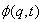IDRSEL(1)=2
scalar=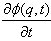IDRSEL(1)=3
scalar=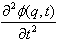Here q represents the variables,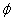represents the constraint,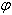represents the constraint when all variables (q) are set to zero, and t represents time.
XMATRX
[double precision]
A 30x30 array that returns the second derivatives of the constraint with respect to the principal axes variables. This is controlled by IDRSEL(3) as follows:
IDRSEL(1)=0
No evaluation.
IDRSEL(1)=1
xmatrx(m,n)=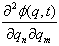Here q represents the variables,represents the constraint, and t represents time.
During the initialization of UCOSUB, UCOVAR and/or UCOMAR should be called to declare the variables.

## Example

def UCOSUB(id, time, q, par, npar, idrsel, iflag):
ORIG_R0X = 11
ORIG_R0Y = 12
ORIG_R0Z = 13

DCMX_U1X = 111
DCMX_U1Y = 112
DCMX_U1Z = 113
DCMX_U2X = 211
DCMX_U2Y = 212
DCMX_U2Z = 213
DCMX_U3X = 311
DCMX_U3Y = 312
DCMX_U3Z = 313

vector = 2*
xmatrx = 155*
if int(par)==5000000:
imars = 2*
ivars = 2*

dir1_id     = int(par)
marker1_id  = int(par)
dir2_id     = int(par)
marker2_id  = int(par)

imars = marker1_id
imars = marker2_id

if dir1_id == 1:
ivars = ORIG_R0X
elif dir1_id == 2:
ivars = ORIG_R0Y
elif dir1_id == 3:
ivars = ORIG_R0Z

if dir2_id == 1:
ivars = ORIG_R0X
elif dir2_id == 2:
ivars = ORIG_R0Y
elif dir2_id == 3:
ivars = ORIG_R0Z

if iflag:
nmar = 2;
nvar = 2;
py_ucomar(id, imars, ivars)
return[0, vector, []]

if idrsel ==1:
scalar = q - q
else:
scalar =0.0

if idrsel ==1:
vector =1.0
vector = -1.0
else:
vector =0.0
vector =0.0
return [scalar, vector, xmatrx]